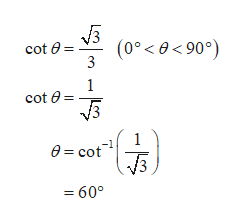# Find the values of θ in degrees (0° < θ < 90°) and radians (0 < θ < π/2) without using a calculator.(a)    cot(θ) = square root of 3 over 3

Question
21 views

Find the values of θ in degrees (0° < θ < 90°) and radians (0 < θ < π/2) without using a calculator.

(a)
cot(θ) = square root of 3 over 3
check_circle

Step 1

Consider the trigonometric equation as cot(θ) = √3/3.

So...help_outlineImage Transcriptionclose(0 90°) cot e 3 1 cot e V3 1 e cot V3 =60° fullscreen

### Want to see the full answer?

See Solution

#### Want to see this answer and more?

Solutions are written by subject experts who are available 24/7. Questions are typically answered within 1 hour.*

See Solution
*Response times may vary by subject and question.
Tagged in

### Trigonometry